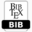AUTHOR(S): Jacob M. Manale TITLE Introducing Smart Symmetries with application to gravity related radiationPDFBIBTEX ABSTRACT Local one-parameter ǫ point symmetries do not always lead to readily integrable results. To address this here, a secondary infinitesimal parameter ω is introduced, leading to what we term smart symmetries. These enable the transformation of the integrals into limit expressions, much like Cauchys contour integration. As proof of validity, we use these type of symmetries to develop a procedure for determining frequencies for detecting any material, depending on the elements constituting it, including metals such as Copper, Silver, Gold and Platinum. Here, we give the frequency for Platinum. KEYWORDS Differential equations, Gravitational waves, Symmetry analysis. REFERENCES  S. Kumei G.W. Bluman. Symmetries and differential equations. Appl. Math. Sci., 81, 1989.  S. Kumei G.W. Bluman. Symmetries and differential equations. Appl. Math. Sci., 81, 1989.  S.C. Anco G.W. Bluman. Symmetry andintegration methods for differential equations. Appl. Math. Sci., 154, 2000.  S. Lie. On integration of a class of linear partial differential equations by means of definite integrals. Arch. Math., 2:328–368 (in German); English transl. published in , 1881.  T. E. Kiess. Exact solutions to einstein’s field equations for perfect spherically symmetric static fluids. Classical Quant. Grav., 26(2):025011, 2009.  L. Kaur and R. K. Gupta. On symmetries and exact solutions of the einsteinmaxwell field equations via the symmetry approach. Phys. Scr., 87(3):035003, 2013.  M. MacCallum C. Hoenselaers H. Stephani, D. Kramer and E. Herlt. Exact Solutions of Einstein’s Field Equations. Cambridge University Press, Cambridge, 2003.  J. M. Manale. New solutions of the heat equation. International Journal of Applied Mathematics and Informatics, 8:15–25, 2014.  T.P. Masebe and J.M. Manale. New symmetries of black-scholes equations (venice, italy, september 2013). In Proceedings of the 2013 International Conference on Applied Mathematics and Computational Methods (AMCM 2013) (Venice, Italy, September 2013), RECENT ADVANCES in APPLIED MATHEMATICS and COMPUTATIONAL METHODS, pages 221–231, Venice, Italy, September 2013. NUAN Press.  Masebe T.P. Adams, C.M. and J.M. Manale. Gaussian type differential equation (interlaken, switzerland february 22-24, 2014). In Proc. Proceedings of the 2014 International Conference on Mathematical Methods, Mathematical Models and Simulation in Science and Engineering (MMSSE 2014) (Interlaken, Switzerland February 22- 24, 2014), RECENT ADVANCES in MATHEMATICAL METHODS, MATHEMATICAL MODELS and SIMULATION in SCIENCE and ENGINEERING, pages 28–31, Interlaken, Switzerland, February 2014. NUAN Press.  J.M. Manale. New solutions of the heat equation and application to thin plate heat conduction (venice, italy, september 2013). In Proc. Proceedings of the 2013 International Conference on Applied Mathematics and Computational Methods (AMCM 2013) (Venice, Italy, September 2013), RECENT ADVANCES in APPLIED MATHEMATICS and COMPUTATIONAL METHODS, pages 90–103, Venice, Italy, September 2013. NUAN Press.  J.M. Manale. Alternative lie type one-parameter point symmetries, with application to gravity related radiation (gdansk, poland, may 15-17, 2014). In Jerzy Balicki, editor, Proceedings of the 2nd International Conference on Mathematical, Computational and Statistical Sciences (MCSS ’14) (Gdansk, Poland, May 15-17, 2014), volume 27 of ADVANCES in APPLIED and PURE MATHEMATICS, Gdansk, Poland, May 2014. WSEAS Press.  J.M. Manale. New symmetry solutions to burgers equation (salerno, italy, june 3-5, 2014). In Proceedings of the 10th International Conference on Applied and Theoretical Mechanics (MECHANICS ’14) (Salerno, Italy, June 3-5, 2014), LATEST TRENDS in APPLIED and THEORETICAL MECHANICS, pages 13–22, Salerno, Italy, 2014. WSEAS Press.  A.P. Arya. Introduction to Classical Mechanics. Pearson Education, London, 2007.  T.W.B. Kibble and F.H. Berkshire. Classical Mechanics. Imperial College Press, Singapore, 2004.  A. Szafer C. Talmadge E. Fischbach, D. Sudarsky and S. H. Aronson. Reanalysis of the eo¨tvo¨s experiment. Phys. Rev. Lett., 56:3660 – 3666, 1986.  H. Zhu W. Zhou Q.S. Wang Z. Zhao W. Jiang Y.C. Liu, X.S. Yang and C.Z Wu. Testing non-newtonian gravitation on a 320 m tower. Phys. Lett. A., 169:131 – 133, 1992.  O.B. Khavroshkin and V.V. Tsyplakov. Geophysical and geochemical fields of pyramids. Proceedings of the first international scientific conference about Bosnian valley of the pyramids, 32:1–41, 2008.  M. Rakhmanov. Dynamics of Laser Interferometric Gravitational Wave Detectors. California Institute of Technology, Pasadena, California, 2000. Cite this paper Jacob M. Manale. (2016) Introducing Smart Symmetries with application to gravity related radiation. International Journal of Mathematical and Computational Methods, 1, 40-47Copyright © 2016 Author(s) retain the copyright of this article.This article is published under the terms of the Creative Commons Attribution License 4.0